# Intermediate Maths solutions for Limits and Continuity Exercise 8(b)

Inter Maths 1B Solutions for Exercise 8(b) Limits and Continuity are given.

These solutions are very easy to understand.

Please study the text book very well.

Observe the example problems and solutions given in the text book. Try them.

Observe the given solutions and try them in your own method.

You can see the solutions for text book Maths 1A

Functions

Exercise 1(a)

Exercise 1(b)

Exercise 1(c)

Mathematical Induction

Exercise 2(a)

Matrices

Exercise 3(a)

Exercise 3(b)

Exercise 3(c)

Exercise 3(d)

Exercise 3(e)

Exercise 3(f)

Exercise 3(g)

Exercise 3(h)

Exercise 3(i)

Exercise 4(a)

Exercise 4(b)

Product of vectors

Exercise 5(a)

Exercise 5(b)

Exercise 5(c)

Trigonometric Ratios up to Transformations

Exercise 6(a)

Exercise 6(b)

Exercise 6(c)

Exercise 6(d)

Exercise 6(e)

Exercise 6(f)

Trigonometric Equations

Exercise 8(a)

Hyperbolic Functions

Exercise 9(a)

Properties of Triangles

Exercise 10(a)

Exercise 10(b)

Inter Maths Trigonometry text book solutions

Maths 1B solutions for

Locus

Exercise 1(a)

Transformation of Axes

Exercise 2(a)

Three Dimensional Coordinates

Exercise 5(a)

Exercise 5(b)

Direction Cosines and Direction Ratios

Exercise 6(a)
Exercise 6(b)
The Plane

Exercise 7(a)

Limits and Continuity

Exercise 8(a)

Exercise 8(b)

Exercise 8(e)

M

# Inter Maths Solutions for Limits and Continuity Exercise 8(b)

Exercise 8(b)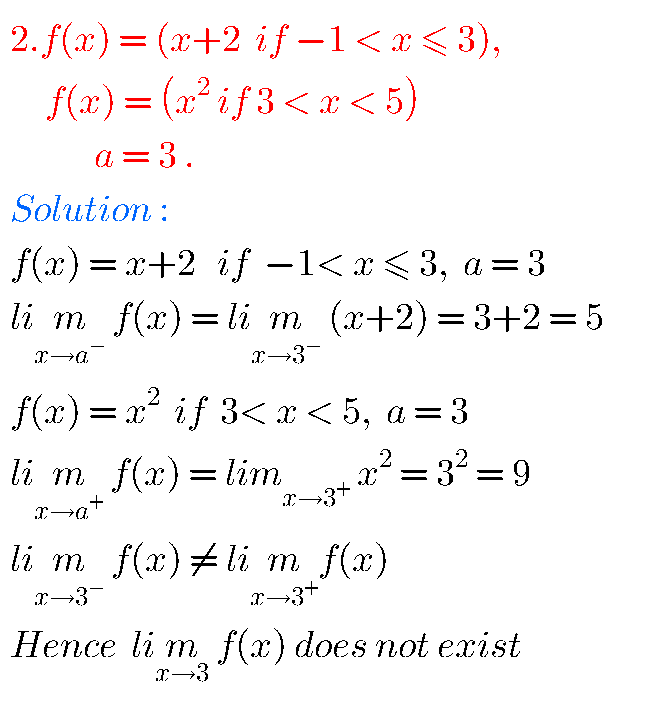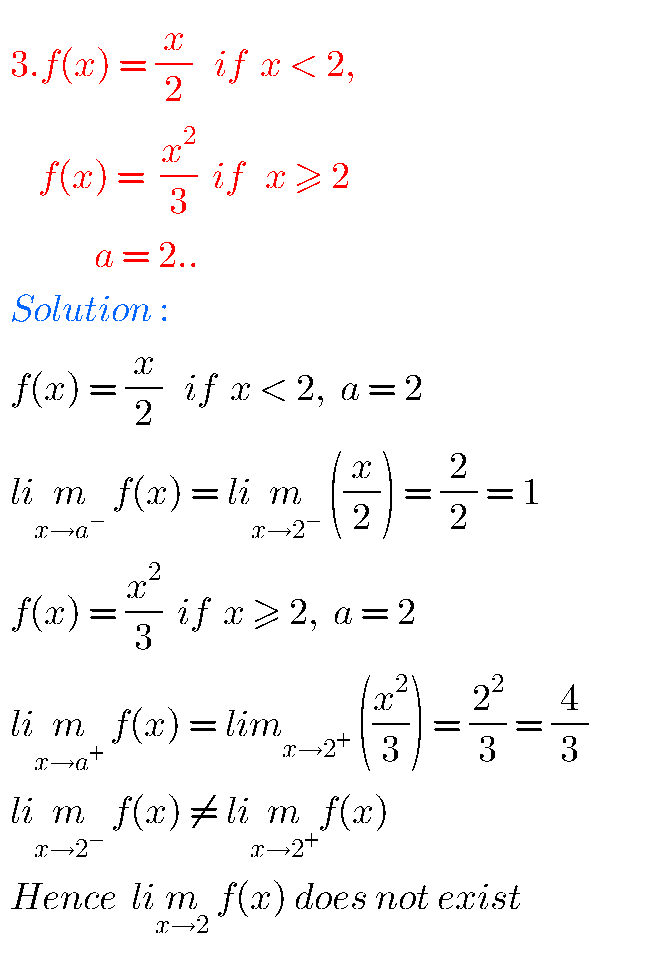## Maths Solutions for Exercise 8(b) Limits and Continuity Inter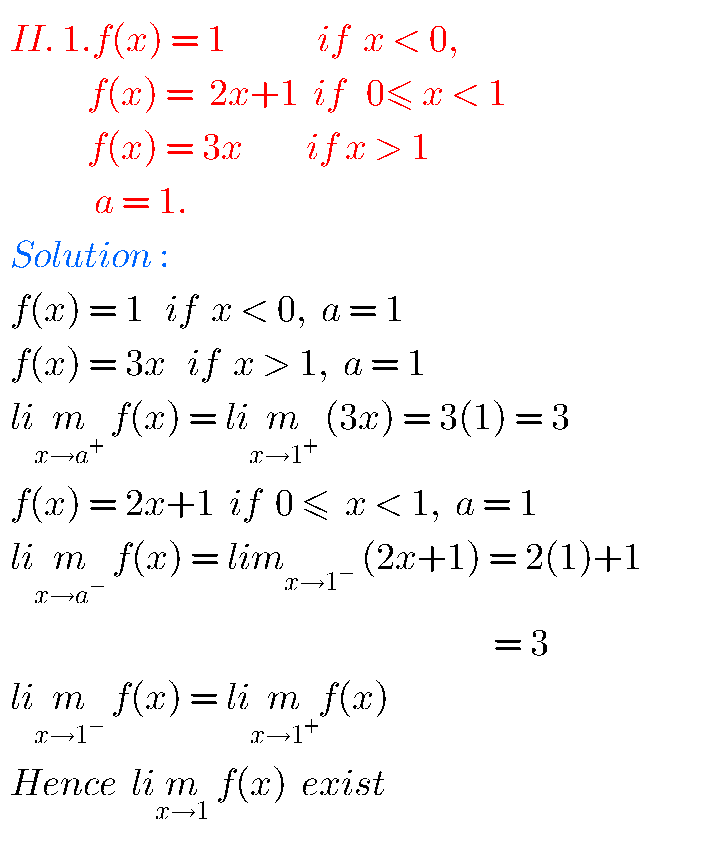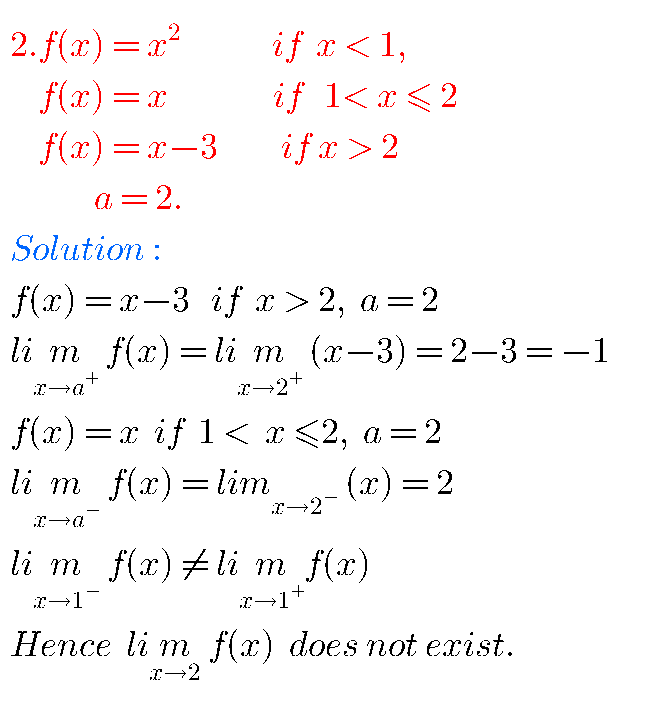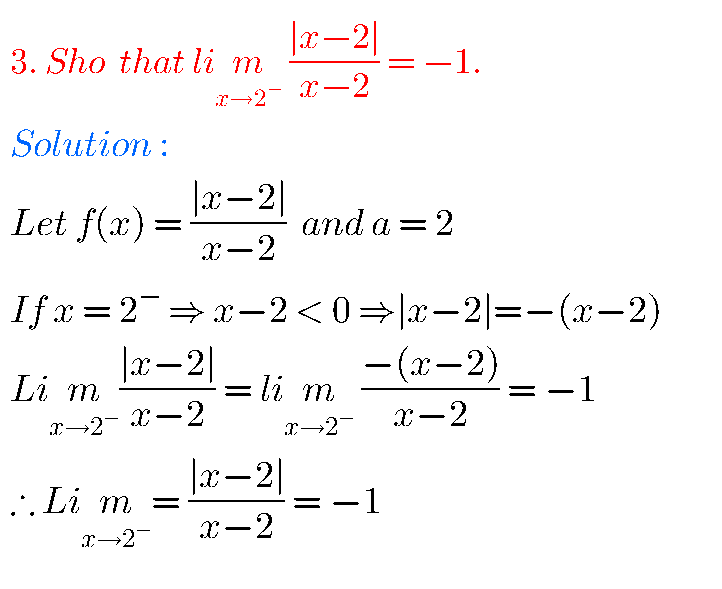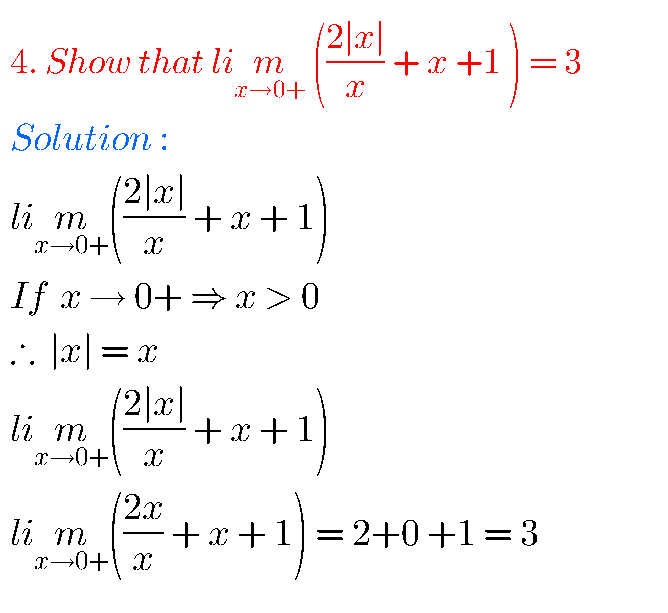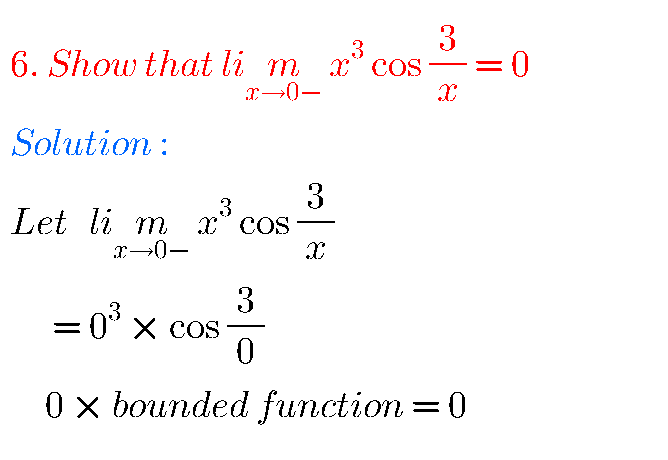## Solutions for Exercise 8(b) Limits and Continuity Maths 1B Inter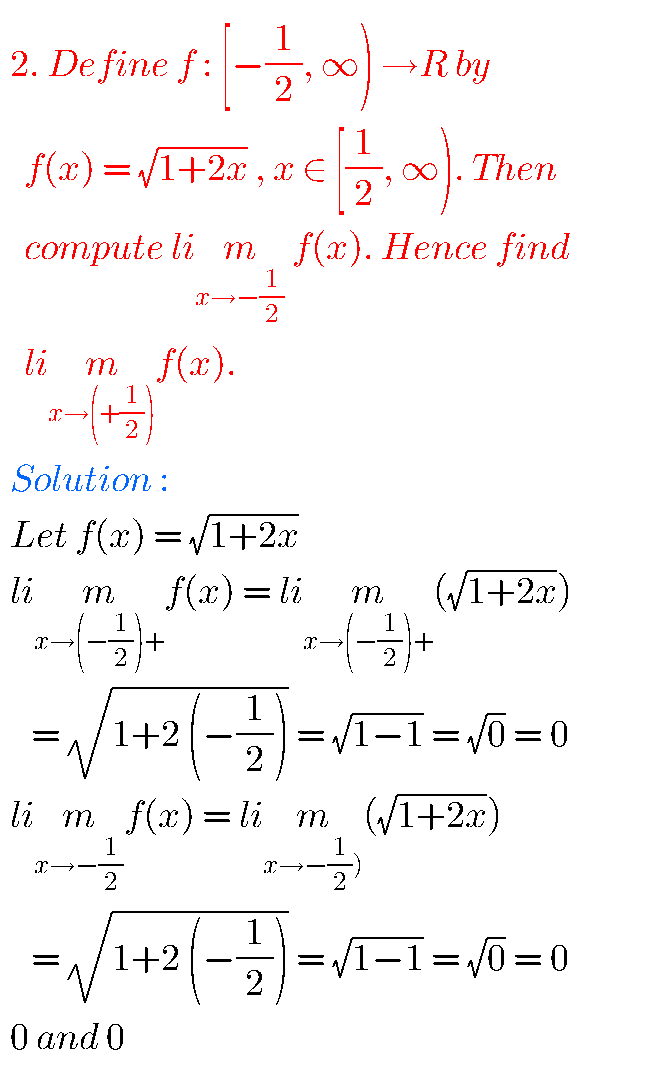Note : Observe the solutions and try them in your own method.

SSC Maths text book Solutions class 10

Inter Maths 1A solutions

Inter Maths 1B solutions

Inter Maths 1IA text book solutions

Inter Maths IIB text book solutions

You can see the solutions for text book Maths 1A

Functions

Exercise 1(a)

Exercise 1(b)

Exercise 1(c)

Mathematical Induction

Exercise 2(a)

Trigonometric Ratios up to Transformations

Exercise 6(a)

Exercise 6(b)

Exercise 6(c)

Exercise 6(d)

Exercise 6(e)

Exercise 6(f)

Trigonometric Equations

Exercise 7(a)

Inverse Trigonometric Equations

Exercise 8(a)

Hyperbolic Functions

Exercise 9(a)

Properties of Triangles

Exercise 10(a)

Exercise 10(b)

Maths 1B solutions for

Transformation of Axes

Exercise 2(a)

Limits and Continuity

Exercise 8(a)

Exercise 8(b)

M